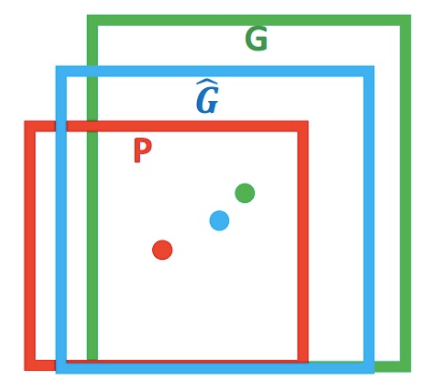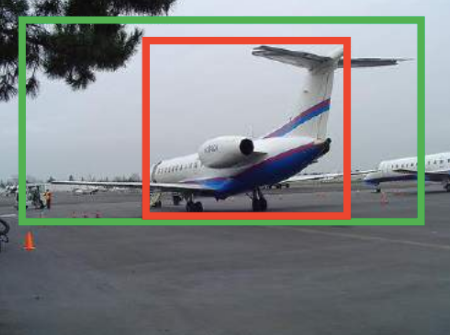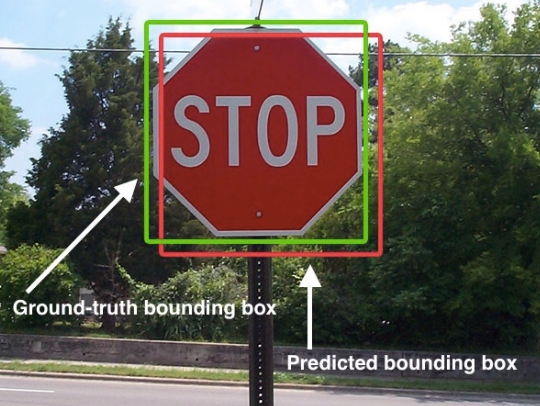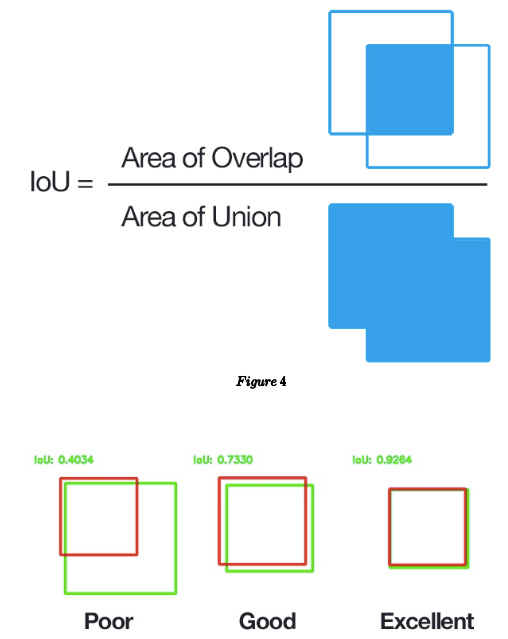# Bounding Box Regression

## Bounding Box Regression

### What is bounding box regression?

Find a $f$ to map the raw input window $P$ to real window $G$, we get $\hat { { G } }$. $f(P) = \hat { { G } }, \hat { { G } } \approx G$Figure 1

### Why we need it?

To learn a transformation that maps a proposed box $P$ to a ground-truth box $G$. You know, if we don’t define something to optimize, we cannot achive the goals.Figure 2

### What is IoU(Intersection over Union)?

Notice the green and red box below.Figure 3

We use Bounding Box Regression to adjust that red window to approach green window.Figure 4

## get IoU according to box parameters
# import the necessary packages
from collections import namedtuple
import numpy as np
import cv2

# define the Detection object
Detection = namedtuple("Detection", ["image_path", "gt", "pred"])

def bb_intersection_over_union(boxA, boxB):
# determine the (x, y)-coordinates of the intersection rectangle
xA = max(boxA, boxB)
yA = max(boxA, boxB)
xB = min(boxA, boxB)
yB = min(boxA, boxB)

# compute the area of intersection rectangle
interArea = (xB - xA) * (yB - yA)

# compute the area of both the prediction and ground-truth
# rectangles
boxAArea = (boxA - boxA) * (boxA - boxA)
boxBArea = (boxB - boxB) * (boxB - boxB)

# compute the intersection over union by taking the intersection
# area and dividing it by the sum of prediction + ground-truth
# areas - the interesection area
iou = interArea / float(boxAArea + boxBArea - interArea)

# return the intersection over union value
return iou


You see, it’s easy to calculate IOU using python.

## How we find that function $\hat{G}$?

### Target mapping $f$:

$\left( P _{ x } , P_ { y } , P _{ w } , P_ { h } \right) = \left( \hat { G } _{ x } , \hat { G }_ { y } , \hat { G } _{ w } , \hat { G }_ { h } \right) ,\left( \hat { G } _{ x } , \hat { G }_ { y } , \hat { G } _{ w } , \hat { G }_ { h } \right) \approx \left( G _{ x } , G_ { y } , G _{ w } , G_ { h } \right)$

### How to map $P$ to $\hat { G }$ in figure 1?

#### Core concept

1. translation
• $( \Delta x , \Delta y ) , \quad \Delta x = P _{ w } d_ { x } ( P ) , \Delta y = P _{ h } d_ { y } ( P )$ \begin{aligned} \hat { G } _{ x } = P_ { w } d _{ x } ( P ) + P_ { x } \\ \hat { G } _{ y } = P_ { h } d _{ y}( P ) + P_ { y } \tag{1} \end{aligned}
2. scaling
• $\left( S _{ w } , S_ { h } \right) , S _{ w } = \exp \left( d_ { w } ( P ) \right) , S _{ h } = \exp \left( d_ { h } ( P ) \right)$ \begin{aligned} \hat { G } _{ w } & = P_ { w } \exp \left( d _{ w } ( P ) \right) \\ \hat { G }_ { h } & = P _{ h } \exp \left( d_ { h } ( P ) \right) \tag{2} \end{aligned}
That means, bounding box regression is to learning $d _{ x } ( P ) , d_ { y } ( P ) , d _{ w } ( P ) , d_ { h } ( P )$, we can find that we could use $d _{ *} ( P ) = \mathbf { w }_{ \star } ^ { \mathrm { T } } \phi _{ 5 } ( P )$ to learn how to map $\phi_ {5}$(pool5 features of proposal P) to $d _{*}$, which is a simple linear regression problem, and we can use the formula $(1)$-$(2)$ to get $\hat {G}$.

$\hat { { G } }$ is forecast value, but we need $\hat { { G } }$ to be $G$. So we still need to find the difference between $G$ and $\hat { { G } }$.

### What’s the difference between $\hat { { G } }$ and $G$?

We want $\hat { { G } }$ to be as close to $G$ as possible, that means, we need to find a bounding box input: features of proposal $\phi _{5}$ (CNN pool5 output) bounding box output: $d _{ x } ( P ) , d_ { y } ( P ) , d _{ w } ( P ) , d_ { h } ( P )$. Then we can map $P$ to $G$.

### How to use the bounding box output to get $G$?

From above we can know that we could get $\hat { { G } }$ from $d _{ x } ( P ) , d_ { y } ( P ) , d _{ w } ( P ) , d_ { h } ( P )$, not $G$. Notice, from $P$ to $G$ means: \begin{aligned} t _{ x } = \left( G_ { x } - P _{ x } \right) / P_ { w } \\ t _{ y } = \left( G_ { y } - P _{ y } \right) / P_ { h }\\ t _{ w } = \log \left( G_ { w } / P _ { w } \right) \\ t _{ h } = \log \left( G_ { h } / P _ { h } \right) \end{aligned}

That means if we reduce the error between $d _{ x } ( P ) , d_ { y } ( P ) , d _{ w } ( P ) , d_ { h } ( P )$ and $t _{ *} = \left( t*_ *{ x } , t _{ y } , t_ { w } , t _{ h } \right) )$, we can really map our $P$ to $G$, because we have bounding box help use to learn the real $t_ {*}$ for us. $\Rightarrow$
We can reduce the loss function: $L o s s = \sum _{ i } ^ { N } \left( t_ { * } ^ { i } - W ^ { T } \phi _ { 5 } \left( P ^ { i } \right) \right) ^ { 2 }$ to accomplish our goal. Here $\phi _ { 5 } \left( P ^ { i } \right)$ means the input to bounding box.

Also to regularize the loss function, we can use: $W _{ * } = \operatorname { argmin }_ { w , } \sum _{ i } ^ { N } \left( t_ { * } ^ { i } - W ^ { T } \phi _{ 5 } \left( P ^ { i } \right) \right) ^ { 2 } + \lambda \left\| \hat { w }_ { * } \right\| ^ { 2 }$ and use gredient descent to get $W$.

### Why we can use $\hat { G } = WP$?

When IoU > $\theta$ (like 0.6), we can think the transformation be a linear transformation, and use that function to adjust. $t _{ w } = \log \left( G_ { w } / P _{ w } \right) = \log \left( \frac { G_ { w } + P _{ w } - P_ { w } } { P _{ w } } \right) = \log \left( 1 + \frac { G_ { w } - P _{ w } } { P_ { w } } \right)$ When $G _{ w } - P_ { w } \approx 0$, we think it as linear. $\lim _ { x = 0 } \log ( 1 + x ) = x$

## Reference

Welcome to share or comment on this post: